# Atoms And Nuclei   Share

## What is Atoms And Nuclei

Everything around us which has mass and occupies space is matter. An atom is the basic unit of matter. It cannot be broken down further using any chemical means because it is the basic building block of an element. Every state of matter solid, liquid, gas, and plasma is composed of either atom either it is neutral (un-ionized), or ionized atoms. An atom is made up of three particles known as protons, neutrons, and electrons. And these particles are also made up from sub-particles. Among these three particles, protons have a positive charge while electrons carry a negative charge and the third particle neutrons have no electrical charge. And the charge of atoms depends on the number of protons and electrons, I.e  An atom is electrically neutral if the number of protons and electrons are equal. And If an atom has more or fewer electrons than protons, then it has an overall negative or positive charge, respectively. These atoms are extremely small or you can say their typical sizes are around 100 picometers. Let's have a look at the below figure.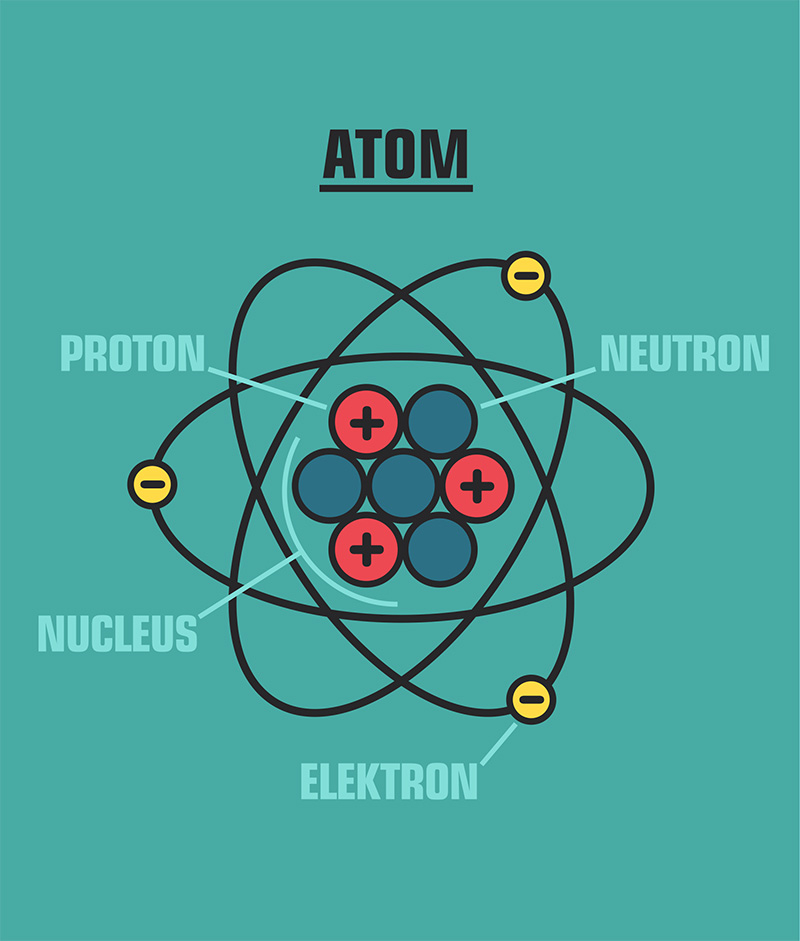From the figure, it is clear that particles like protons and neutrons are situated at the center of the atom while the electrons are revolving in orbit around this center.

So the dense region consisting of protons and neutrons at the center of an atom in the figure is known as The atomic nucleus of an atom. Every atom is composed of such nucleus and some elections will be surrounding it, bound together by electrostatic force. Studying these atoms and Nuclei will help us to have a thorough understanding of matter. Studying about the nucleus and its reactions will help us to understand more about nuclear energy, which is a very useful renewable energy. That's why it is very important to study about Atoms and Nuclei.

Atoms and Nuclei is one of the most important chapters from modern physics while preparing for all competitive exam because it helps you to understand atoms which are the basic unit of matter. This is easy to understand and a high scoring topic. The Concept of Atoms and Nuclei and some other chapters of physics are mixed in miscellaneous questions which are asked in various competitive exams.

Prepare Online for JEE Main/NEET

Crack JEE 2021 with JEE/NEET Online Preparation Program

## Notes on the Atoms And Nuclei

So we will discuss step by step about important topics from this chapter followed by an overview of this chapter. Then we will understand important formulas from this chapter. Remembering these formulas will increase your speed while question-solving.

## Atoms And Nuclei Topics

• Alpha-particle scattering experiment; Rutherford's model of atom;

• Bohr model, energy levels, hydrogen spectrum.

• Nucleus-Composition and size, atomic masses, isotopes, isobars; isotones.

• Radioactivity, emission of alpha, beta and gamma particles/rays, and their properties; radioactive decay law.

• Mass-energy relation, mass defect; binding energy per nucleon and its variation with mass number, nuclear fission, and fusion.

## Atoms And Nuclei Topics  Overview

In Atoms And Nuclei chapter you will study about basic of atoms and basic of nucleus inside the atom. To study about atom various scientists perform various experiments and suggest various models of an atom with some explanation.

For example, Thomson gives the "plum pudding" model in which he said the atom consists of a positive material known as "pudding" with some negative materials  ("plums") distributed throughout. Later, famous scientist, Rutherford gives Rutherford's model of the atom after performing an Alpha Particle scattering experiment.

So in this chapter, you will learn about

• Rutherford's model of the atom- Rutherford conducted an experiment in which a thin sheet of gold was bombarded with α-particles. In this experiment, he studied the trajectory of the α-particles after interaction with the thin sheet of gold. Based on the observations and the result of the experiment various conclusion were made about properties about an atom. Please have a look at observations of the experiments about the path of α-particles after interaction with the thin sheet of gold as shown in the figure.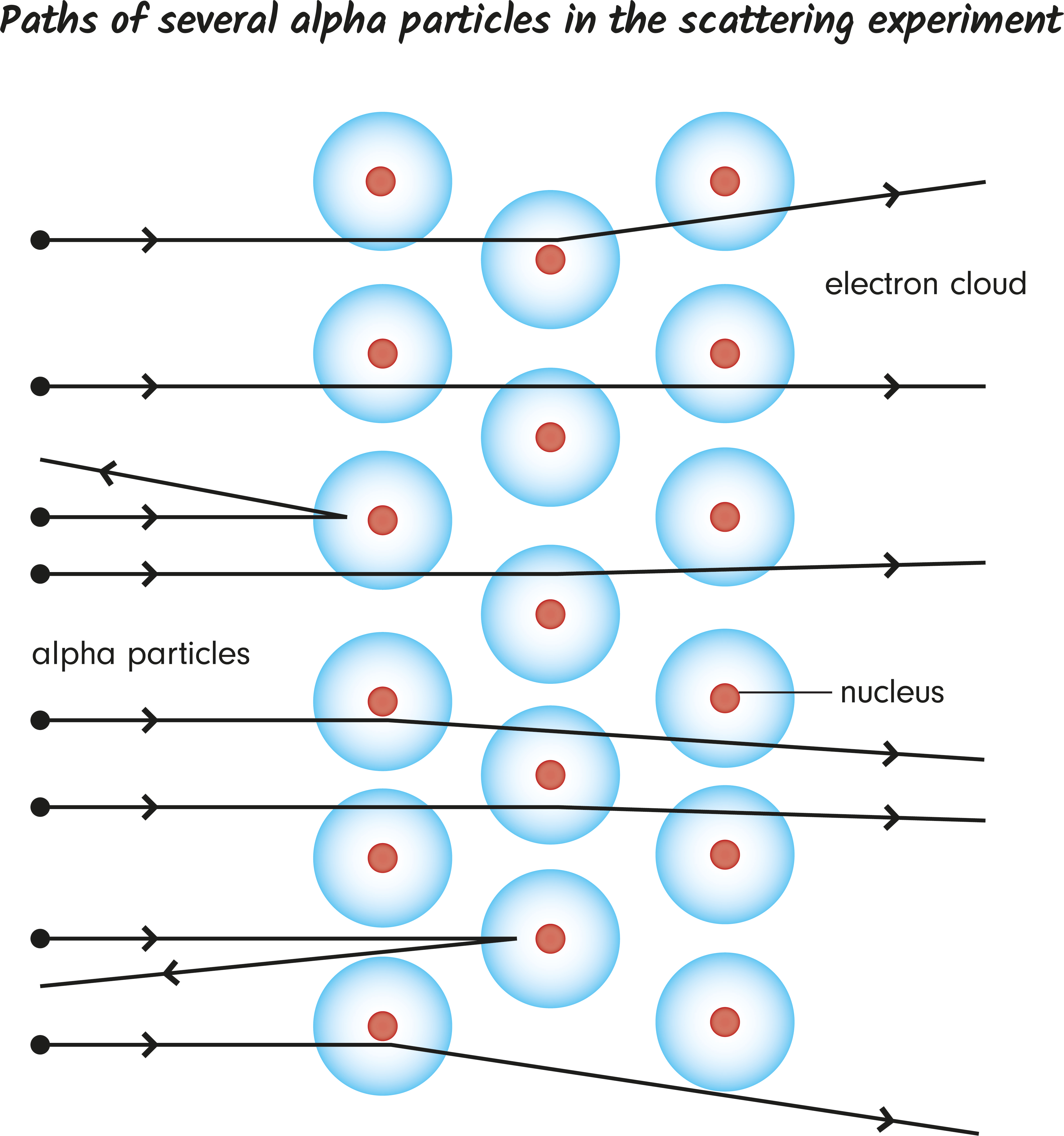• Bohr Model-

This model is a modification of the earlier Rutherford Model. According to this model, an atom consists of a small, positively-charged nucleus and negatively-charged electrons orbiting around it in an orbital. These orbital can have different sizes, energy, etc. And the energy of the orbit is also related to its size, I.e The lowest energy is found in the smallest orbit. So if the electron is orbiting in nth orbit then we will study about its Velocity in nth orbital, Radius of nth orbital, Energy of electron in nth orbit, etc. Energy is also emitted due to the transition of electrons from one orbit to another orbit. This energy is emitted in the form of photons with different wavelengths. This wavelength is given by the Rydberg formula. You will also about Bohr Model of Hydrogen atom. When electrons make transitions between two energy levels in an atom various spectral lines. The emission spectrum of the hydrogen atom has been divided into various spectral series like Lyman series, Balmer series, Paschen Series Etc.

• Nucleus- You will learn about various characteristics of the nucleus, like the composition of the nucleus, size of nucleus, etc. You will also learn some terminology about elements like its atomic mass, its atomic number. You will also learn about isotopes, isobars; isotones.

• Nuclear reactions- You will learn about important concepts like Mass-energy relation, mass defect, Nuclear Binding Energy, etc. Nuclear Binding Energy is the minimum energy which is required to separate the nucleons up to an infinite distance from the nucleus. The graph binding energy per nucleon will explain clearly how binding energy varies with mass number. You will also learn about Nuclear Fission and Nuclear Fusion. Nuclear Fission is the process of the splitting of a heavy nucleus into two or more lighter nuclei while Nuclear Fusion is the process of combining two lighter nuclei to form one heavy nucleus.

Understanding all these concepts will help you to understand atoms and nuclei chapter.

## Atoms and Nuclei Formulas

• Bohr quantization principle-

• The radius of nth orbital

• The velocity of the electron in nth orbital-

• The energy of an electron in nth orbit-

• The relation between K.E., P.E. & total energy

• The energy emitted due to the transition of electron-

• Ionization energy-

• Moseley's law-

• Bragg's law

• Energy-mass equivalence-

• Binding energy-

• For α -decay-

• Number of nuclei in terms of half-life

• Half-Life Time

## How to prepare for Atoms and Nuclei

First, you should have good command over the concepts of Atoms and Nuclei and you should also know how to apply them well at the time of the entrance exam or while solving questions. Please try to understand each concept from this chapter, with the help of theory, questions with solutions and video lectures on each important concept. For each concept practice enough problems so that you have a thorough understanding of the concept. Solve all the questions at home with proper concentration and try to do all calculation by yourself without seeing the solution first. Concepts like  Bohr quantization principle, Energy emitted due to the transition of electrons, graphs of Binding energy per nucleon, Law of radioactivity, etc are very important with respect to various competitive exams. Remember all the formulas of calculating Radius, Velocity, Energy of electron in nth orbit. This will help you while solving questions. Practice problem on calculating the Q value of reactions.

## Atoms and Nuclei Tips

• Make a plan to prepare for this chapter and Stick to a Timetable.
• Study the concept first and then start solving questions. Don't go through question directly without knowing the concept.
• Formulas from this chapter are very important from the point of view of competitive exam. So please remember them and solve lots of questions based on these formulas.
• Solve previous year question of various exam from this chapter. Find out what mistakes you are doing while solving it. Learn from these mistakes and try to not repeat those mistakes.• Use smart methods to solve questions.

## Atoms and Nuclei Books

For Atoms and Nuclei, chapter concepts in NCERT are enough but you will have to practice lots of questions including previous year questions and you can follow other standard books available for competitive exam preparation like Concepts of Physics (H. C. Verma) and Understanding Physics by D. C. Pandey (Arihant Publications).

## Physics Chapter wise Notes For Engineering and Medical Exams

 Chapters No. Chapters Name Chapter 1 Physics and Measurement Chapter 2 Kinematics Chapter 3 Laws of motion Chapter 4 Work Energy and Power Chapter 5 Rotational Motion Chapter 6 Gravitation Chapter 7 Properties of Solids and Liquids Chapter 8 Kinetic theory of Gases Chapter 9 Thermodynamics Chapter 10 Oscillations and Waves Chapter 11 Electrostatics Chapter 12 Current Electricity Chapter 13 Magnetic Effects of Current and Magnetism Chapter 14 Electromagnetic Induction and Alternating currents Chapter 15 Electromagnetic Waves Chapter 16 Optics Chapter 17 Dual Nature of Matter and Radiation Chapter 19 Electronic devices Chapter 20 Communication Systems Chapter 21 Experimental skills

### Topics from Atoms And Nuclei

• Bohr model, energy levels, hydrogen spectrum. ( JEE Main, COMEDK UGET, KEAM ) (18 concepts)
• Radioactivity-alpha, beta and gamma particles/rays and their properties ( JEE Main, COMEDK UGET, KEAM ) (8 concepts)
• Mass-energy relation, mass defect; ( JEE Main, COMEDK UGET, KEAM ) (6 concepts)
• radioactive decay law. ( JEE Main, COMEDK UGET, KEAM ) (12 concepts)
• nuclear fission, and fusion. ( JEE Main, COMEDK UGET, KEAM ) (5 concepts)
• Rutherford's model of atom ( JEE Main, COMEDK UGET, KEAM ) (3 concepts)
• Bohr's model ( JEE Main, COMEDK UGET, KEAM ) (6 concepts)
• Nuclei ( JEE Main, COMEDK UGET, KEAM ) (6 concepts)
• Radioactivity ( JEE Main, COMEDK UGET, KEAM ) (4 concepts)
• X-rays ( JEE Main, COMEDK UGET, KEAM ) (5 concepts)
• Nuclear reaction ( JEE Main, COMEDK UGET, KEAM ) (2 concepts)

### Important Books for Atoms And Nuclei

•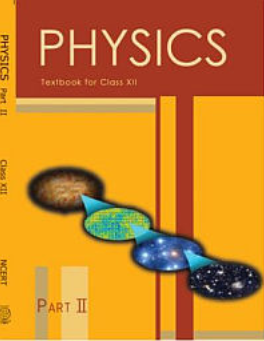•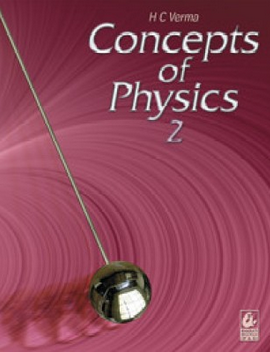•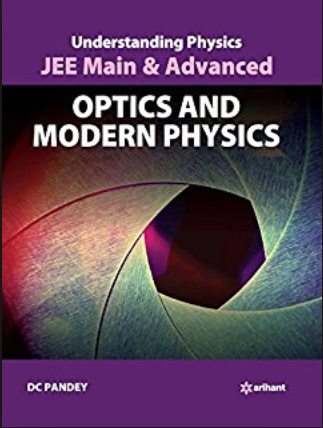Exams
Articles
Questions